Chapter 5 Eigenvalue and Eigenvector II

5.1 Invariance Subspace

F : ( C or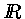) a field

<Def> A subspace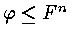is invariant ( of A )

if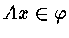, where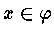Example : 1. If v is Eigenvector of A <v> the eigenspace of A

then <v> is invariant .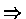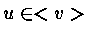u = c u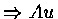=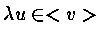2.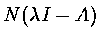: the null space of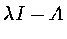<Thm 5.5.1>

Let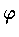be a subspace of Fn with basis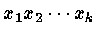Thus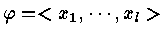LetThenis invariant under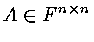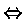there exist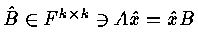Recall that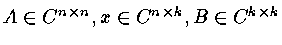Ax = xB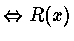is invariant and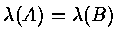Schur's Thm :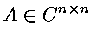,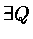unitary s.t. Q* A Q = T = D + N

Real Shur's Thm :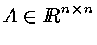,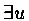orthogonal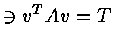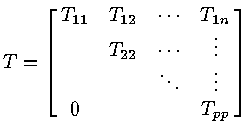where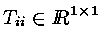or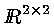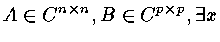s.t. if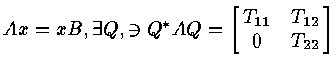and<Thm 5.1.2 > Letbe invariant underand Let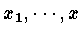be a basis forLet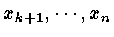be any n - k vectors s.t.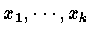is a basis for Fn . Let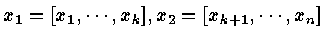and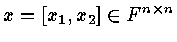Define B = x-1 A x , then B is block upper triangular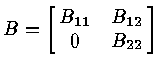Furthermore A x1 = x1 B

(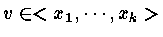, xv ==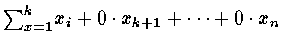)

Note : 1.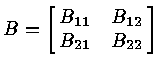If B21 = 0 , thenis invariant 2.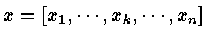can be a orthogonal matrix

Exercise 5.1.10 : Ifis invariant under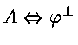is invariant under A*

Note : If A is Hermitian ( A* = A ) , then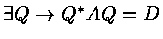[Real Schur's Thm]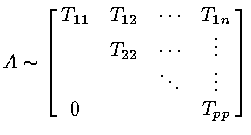orNote : 1. If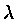is an Eigenvalue of A , then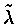is also an Eigenvalue of A 2. v = v1 + i v2 is the Eigenvector of A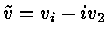is also the Eigenvector of A